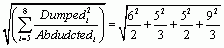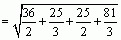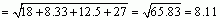# Jig:Saw’s puzzle solutions

## Puzzle 1

Describe the main sections of a research report.

• Abstract: A short (approx. 200 words) summary of the whole study.

• Introduction: The introduction is the ‘why’ part of the paper, i.e., why the experiment is needed. It should discuss past research that has led to the researcher’s current hypothesis. It might describe how previous findings are inconsistent or ambiguous and how the current research will clarify the situation. The introduction normally moves from the general to the specific: it begins with some general context about the research and then discusses specific studies. It usually finishes by saying what the hypotheses of the current experiment are.

• Method: A description of how the research was done. There should be enough detail that someone else could repeat the research. It’s common to split this section up into who took part (participants), what tasks were used (measures/materials), special equipment that was used (apparatus), the type of research design that was used (design), a description of what happened (procedure), and how the measures were scored (scoring). It’s unusual not to see participants and procedure, but the other sections may or may not be there.

• Results: This is where the authors describe what they found. It normally starts with some descriptive statistics about the sample, and then moves on to the inferential statistics. The inferential statistics are used to test the hypotheses.

• Discussion: In this section the author(s) use past research and theories to try to explain their findings. The discussion usually begins with a summary of what was found, then moves on to discuss what this means for their theory (hypotheses) and the real world, and then ends with a list of the limitations of the study and what still needs to be done.

• References: The reference list appears at the end of the paper. It is important because it provides a list of all the sources used in the paper so that the reader can easily look up all of the research papers that are cited in the text. If an article is cited in a paper, it must appear in the reference list. Conversely, if a source appears in the reference list, it must be cited somewhere in the paper.

## Puzzle 2

What is the main difference between qualitative and quantitative research?

Qualitative research gathers evidence for a theory from what people say or write. Quantitative research, on the other hand, gathers evidence for a theory through measurement of variables that produce numeric outcomes.

## Puzzle 3

Look at the variables in Figure 2.2 (in the book) and complete Table 2.3 (in the book).

Table 2.3 (completed): Table to classify variables from Figure 2.2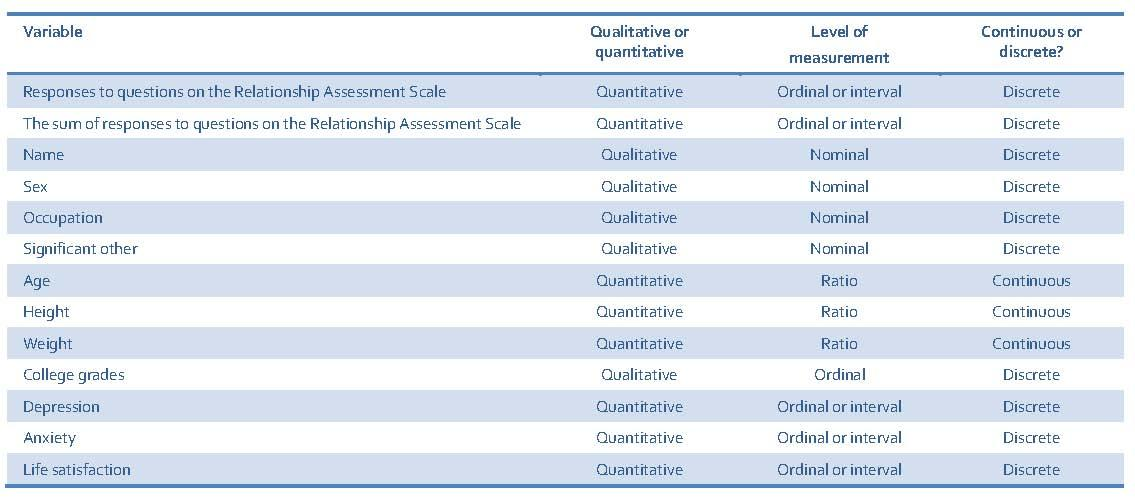## Puzzle 4

After his conversation with Alice, Zach was wondering how he could sell more of his band’s T-shirts. He found an article claiming that sales could be estimated from the price of the T-shirt and how sick the fans thought the design was (from 0 = totally lame, to 10 = totally on trend):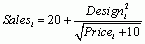Use the equation above and the values in Table 2.4 (in the book) to calculate the sales that would be generated from each combination of price and design. Complete the final column of the table.

Your completed table should look like the one below, but I will go through how I got the first answer.

Table 2.4 (completed): Possible selling price and design ratings of T-shirts.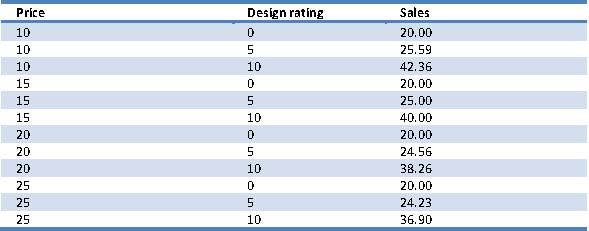To calculate the sales that would be generated from a price of 10 and a design rating of 0 (totally lame), we could first input these values into the equation so that it becomes:BODMAS tells us to calculate everything in brackets first, and although there aren’t any brackets in this equation, brackets around 10 + 10 are implicit because the square root symbol covers both values, so we would do this addition first: 10 + 10 =20.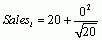Next we move onto ‘order’, so we would calculatewhich is 0 and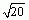which = 4.47, and the equation becomes: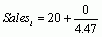Then we would do the division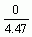:Therefore, the total number of sales generated from a price of 10 and a design rating of 0 would be 20 T-shirts.

## Puzzle 5

Calculate: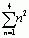The 4 at the top of the summation symbol is the stopping point, or the upper limit of summation. The n = 1 is the starting point, or the lower limit of summation. Therefore, we start at n = 1 and stop at n = 4. The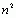means ‘square each value before adding them up’: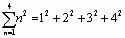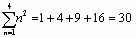## Puzzle 6

Calculate: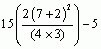First we deal with everything in brackets. We start with the big brackets, but to do the stuff in those, we need to apply BODMAS to everything within those brackets first. So, we start with the small brackets first, so that is 7 + 2 = 9 and 4 × 3 = 12:Next do the orders or exponents. So, take the 92 and change that to 81: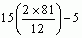Then look for any multiplication or division. There is one of each: there’s a 2 ´ 81 and also a divide by 12. As they are part of the same thing, it makes sense to do the 2 ´ 81 first because that’s what gets divided by 12. So we can replace the 2 ´ 81 with 162: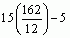and then divide this value by 12, which gives 13.5: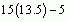Now we have dealt with the big brackets, we can look again for orders (there aren’t any) so we move onto division/multiplication. There is a multiplication: 15 × 13.5, which gives 202.5. Finally, we do the subtraction; 202.5 – 5 = 197.5, so the final answer is 197.5: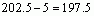## Puzzle 7

Calculate: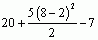First we deal with the brackets, so that is 8 − 2 = 6, so change (8 −2) to 6: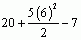Next do the orders or exponents. So, take the 62 and change that to 36: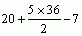Then look for any multiplication or division. There is one of each: there’s a 5 ´ 36 and also a divide by 2. As they are part of the same thing, it makes sense to do the 5 ´ 36 first because that’s what gets divided by 2. So we can replace the 5 ´ 36 with 180:Then divide this value by 2, which gives 90. Finally, we deal with any addition and subtraction, and we do this from left to right, so that gives 20 + 90, which is 110, and then subtract 7 from it. So, the answer is 103: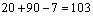## Puzzle 8

Zach measured 10 people’s mood score out of 10 (0 = worst ever mood, 10 = best ever mood) after one of his band’s gigs (Table 2.5 in the book and reproduced below). Use the values in the table to calculate:Table 2.5 (reproduced): Mood scores after a Reality Enigma gig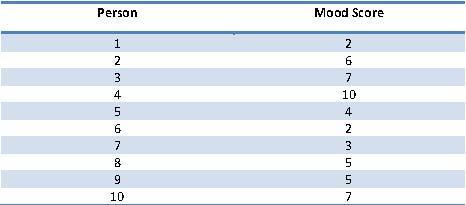Let’s first unpick what the first equation is asking us to do:The i = 2 at the bottom of the sigma symbol means start at person 2 and the n at the top of the symbol tells us to keep adding the scores until we reach the last person’s score. The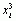means that we need to cube each person’s score before adding them up: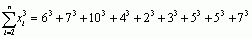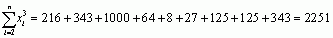So the answer is 2251. Next, we unpick what the second equation is asking us to do: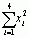The i = 1 at the bottom of the sigma symbol means start adding at person 1 and the 4 at the top of the symbol means keep adding until we reach person 4. Themeans that we need to square each person’s score before adding them up: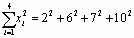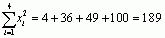## Puzzle 9

Table 2.6 (in the book and reproduced below) shows the average minutes per day that 10 Chippers spend on memoryBank looking at other people’s lives. Using the scores in the table and remembering to use BODMAS, calculate:Table 2.6 (reproduced): Minutes per day spent on memoryBankThe sigma symbol is in the brackets and we have to deal with the brackets first, so that means we’d sum the scores first, starting at person 4 (because of the i = 4 at the bottom of the symbol), and finishing at the last person’s score (because of the n at the top of the sigma). Having done that, we’d deal with the square root that is outside of the brackets, so basically we need to add the scores and then take the square root of the total: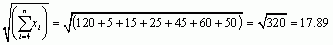## Puzzle 10

Nick asked fans on memoryBank to rate how likely they thought it was the Alice had been abducted and, also, how likely they thought it was that Alice had dumped Zach. Ratings were from 0 = not at all likely, to 10 = certain (Table 2.7 in the book and reproduced below). Use the scores in the table and remembering to use BODMAS, calculate: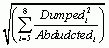Table 2.7 (reproduced): Likelihood (out of 10) of Alice having been abducted vs. her having dumped Zach, as rated by 10 fans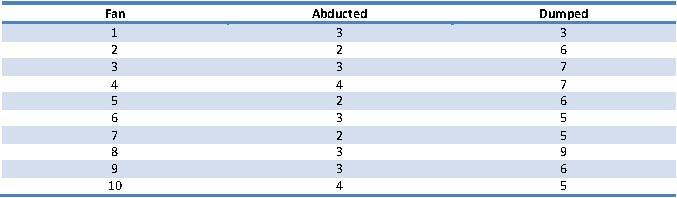The equation is telling us that, starting from the 5th score and finishing at the 8th score, we need to take each dumped score, square it, and then divide each of these squared dumped scores by the corresponding abducted score, and then add them up. We then take the square root of the final score. Here are my workings: